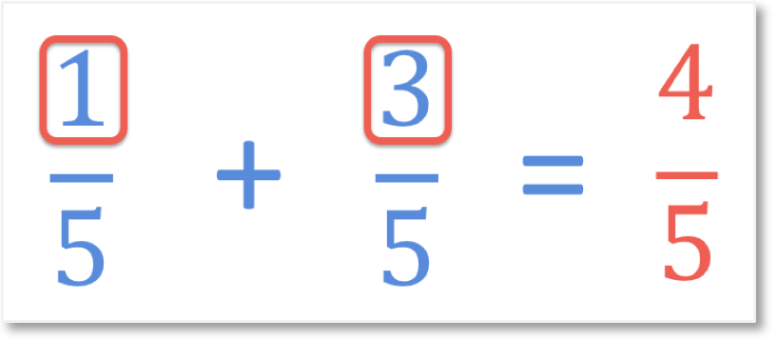# Shading and Identifying Fractions of Shapes

Shading and Identifying Fractions of Shapes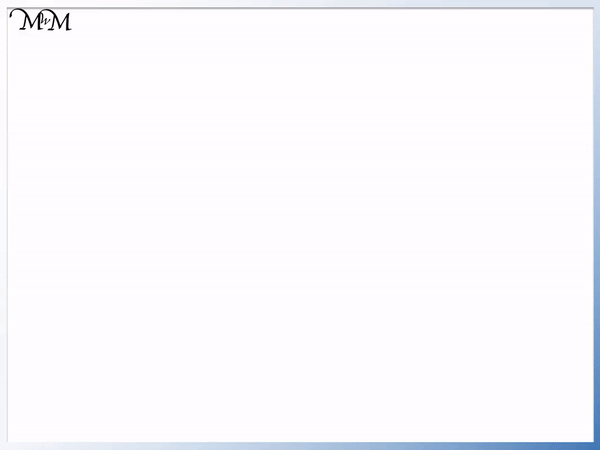• The number on the bottom of the fraction, below the dividing line is called the denominator.
• The denominator tells us how many equal parts to divide the shape into.
• The denominator is two, therefore the shape is divided into two equal parts.
• The number on the top of the fraction, above the dividing line is called the numerator.
• The numerator tells us how many of the equal parts are shaded in.
• The numerator is one and therefore only one part is shaded in.
• We can read the fraction 'one half' as 'one out of two'.
• This means that 'one out of two' equal parts are shaded in.
The denominator of our fraction tells us how many equal parts to divide the shape into.

The numerator of the fraction tells us how many of these parts to shade.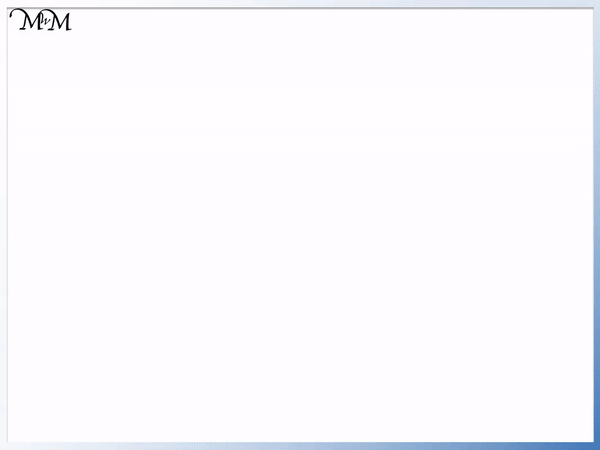• Our fraction is   2 / 3.
• The denominator of three means that we will divide our shade into three equal parts.
• The numerator of two means that we will shade two of these three parts.
• It does not matter which two out of the three parts are shaded in.Supporting Lessons

#### Identifying the Fraction of the Shape that is Shaded# Fractions of Shapes

## What are the Parts of a Fraction

There are two parts of every fraction:

1. The numerator is the number on the top of the fraction, above the dividing line.
2. The denominator is the number on the bottom of the fraction, below the dividing line.For example in the fraction   1 / 2  , the numerator is 1 and the denominator is 2.A fraction is a given amount out of a total. Fractions tell us how many equal parts an amount has been divided into and how many of these parts we have.

The denominator on the bottom of a fraction tells us how many equal parts a shape has been divided into.

The numerator on the top of a fraction tells us how many of these parts we have.

In the fraction   1 / 2  , the denominator of 2 tells us that the shape is divided into 2 equal parts.

The numerator of 1 then tells us to shade in 1 of these 2 parts of the shape.The three parts of a fraction are the numerator on top, the denominator on the bottom and the dividing line which separates them.

The fraction of   1 / 2   means that the shape is divided into 2 equal parts and only 1 of these 2 parts is shaded.The dividing line of a fraction can be read as meaning 'out of'. For example,   1 / 2   can be read as 1 part out of 2.

The dividing line of a fraction means to divide the number above it by the number below it.   1 / 2   means 1 ÷ 2. One whole divided into 2 equal parts.

## How to Shade Fractions of Shapes

1. Read the denominator, which is the number on the bottom of the fraction.
2. Divide the whole shape into this many equally sized parts.
3. Read the numerator, which is the number on the top of the fraction.
4. Shade in this many of the equally sized parts.

For example, here is the example of shading the fraction   2 / 3

In this question we are going to shade two thirds of this whole rectangle.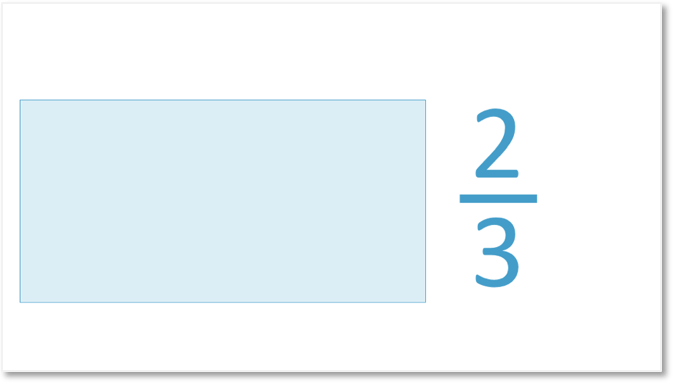The first step is to read the denominator on the bottom of the fraction. The denominator is 3.

This means that the rectangle will be divided into 3 equally sized parts.

To divide a shape into a given numbers of parts, use one less line than parts required. So to divide a shape into 3 parts, only 2 lines are needed.The next step is to read the numerator on the top of the fraction. The numerator is 2.

This means that 2 of these 3 parts will be shaded in.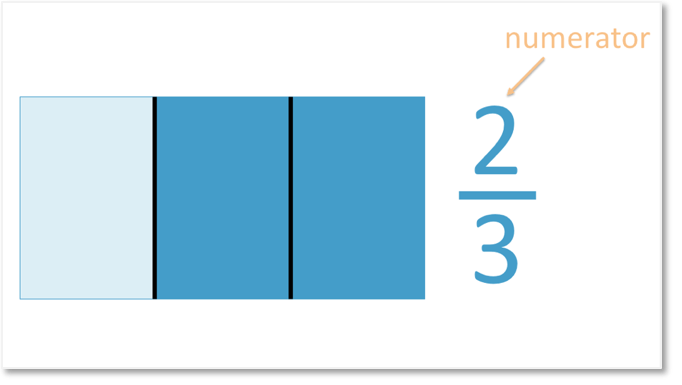It does not matter which parts are shaded in when shading a fraction of a shape. The fraction   2 / 3   simply tells us to shade any 2 of the 3 parts in.Two thirds of the rectangle is shaded.

Here is another example of colouring a given fraction. We have the question of   1 / 4  .The fraction   1 / 4   means that one out of four equal parts is to be shaded.

The denominator is 4, so the rectangle is divided into 4 equal parts.

The numerator is 1, so 1 of the 4 equal parts is shaded in.

It does not matter which of these 4 parts is shaded in. You can pick any part you like.

Most typical shading fractions questions will already show shapes divided into equal parts and ask you to shade the correct number in. If so, then the numerator on the top of the fraction tells you how many to shade.

Here is another example of drawing the fraction   5 / 6  . This means that 5 out of 6 equal parts are shaded in.The first step is to read the denominator on the bottom, which is 6. The shape is divided into 6 equal parts.

The next step is to read the numerator on top, which is 5. 5 of the 6 parts are shaded in.

6 / 6   would have been 6 out of 6, which is the whole shape. When shading in   5 / 6  , there will be 5 parts shaded in, which means that there will be one part that is not shaded in. It does not matter which of the 6 parts you have chosen to not shade in here.

There are several possible answers when asked to colour in a fraction of a shape because you can choose different parts to shade in each time.

Here are just some alternative answers for colouring the fraction   5 / 6  .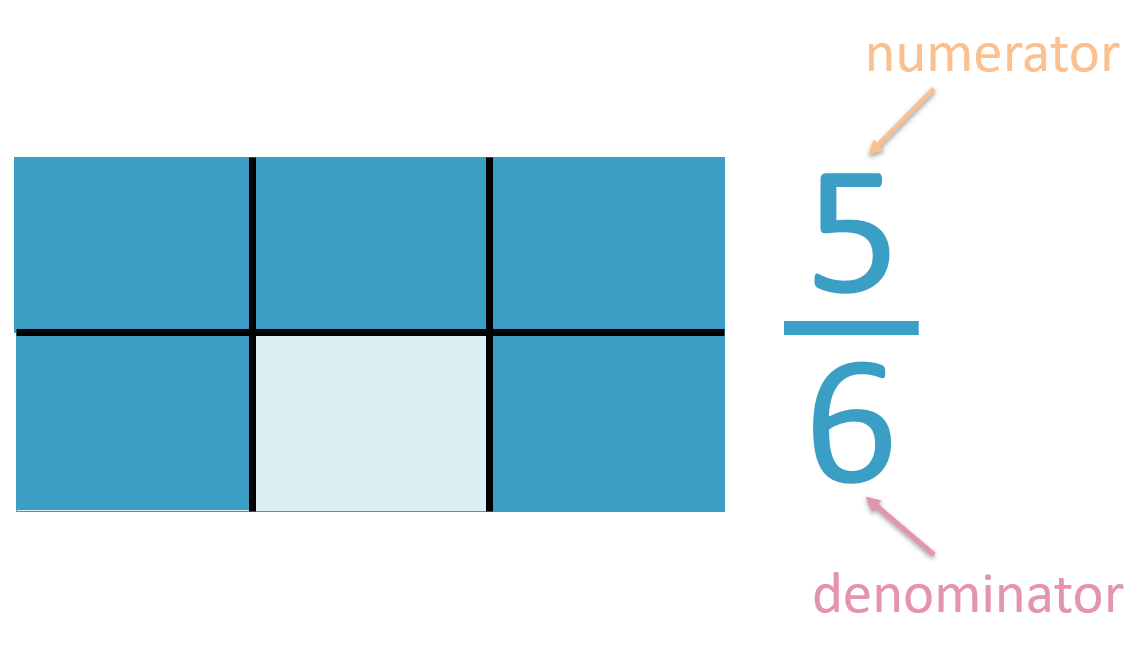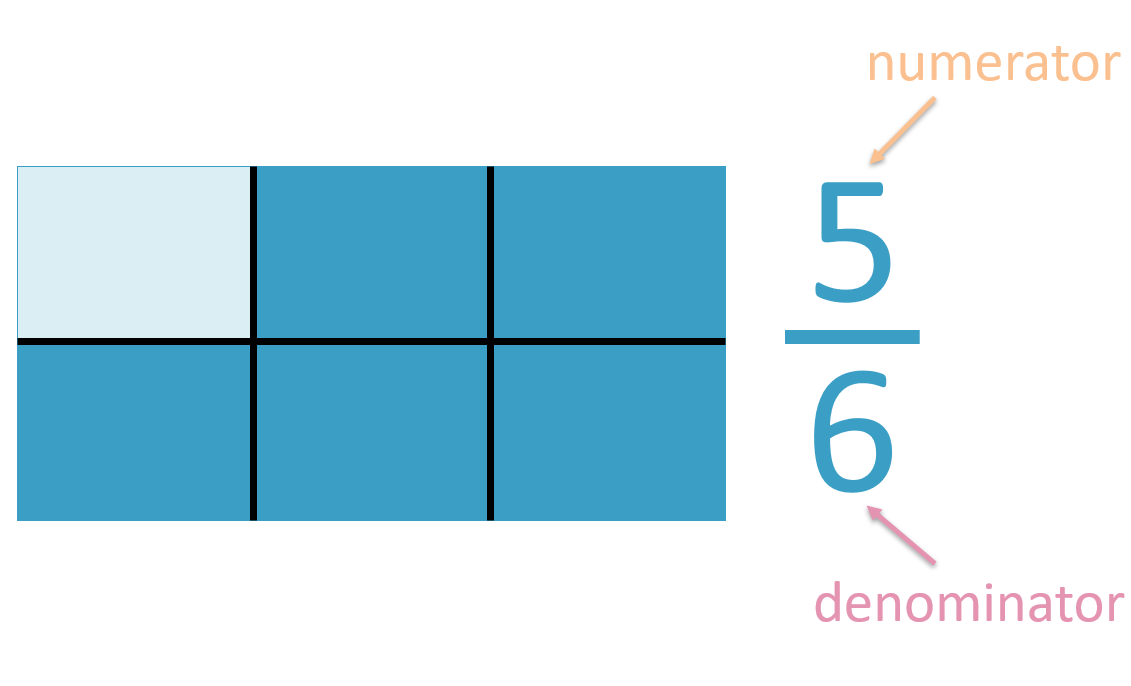## What is the Fraction Shaded?

1. Count the total number of parts the shape is divided into.
2. This number is the denominator and is written below the dividing line of the fraction.
3. Count the number of parts that are shaded in.
4. This number is the numerator and is written above the dividing line of the fraction.

For example, here is a fraction showing one out of 3 parts shaded in. We are asked to fill in the blanks of the fraction in this question.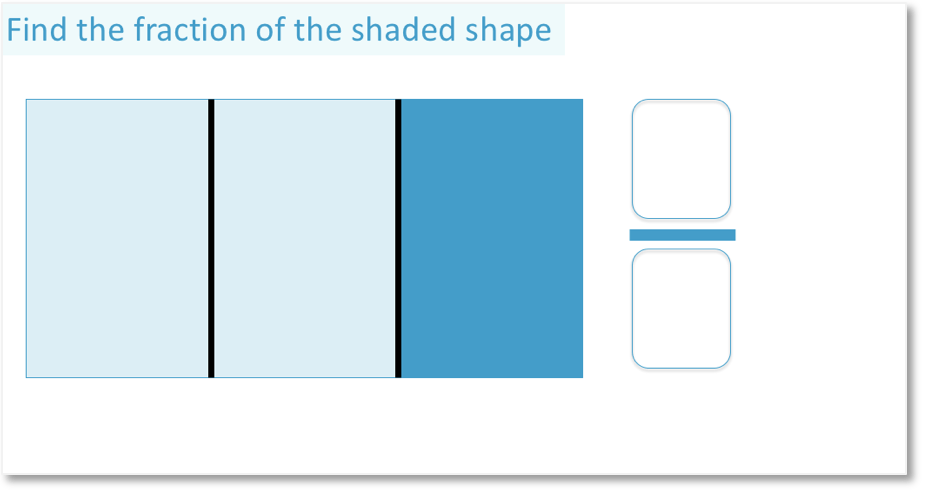The first step is to count the total number of equal parts that the shape is divided into.

This shape is divided into 3 equal parts in total, so we write a 3 on the bottom of the fraction. 3 is the denominator of the fraction.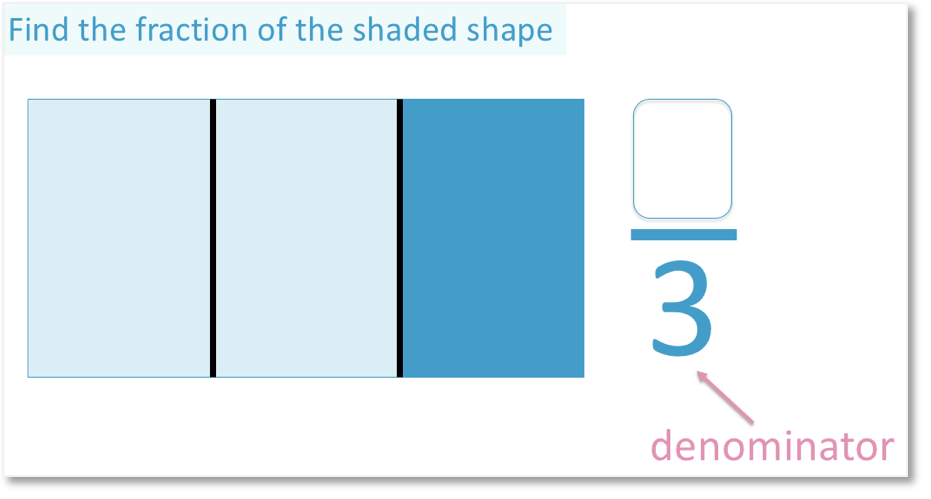The next step is to count the number of parts that are shaded.

Only 1 part of this rectangle is shaded. We write a 1 on the top of the fraction as the numerator.

We have a horizontal dividing line separating the two numbers in a fraction.The fraction of the shaded shape shown is   1 / 3  , which means one out of 3.

We have coloured in 1 of the 3 parts of this shape.

Here is an example of dientifying the fraction shown. There are 3 parts shaded in out of 4 parts in total. One part is not shaded in.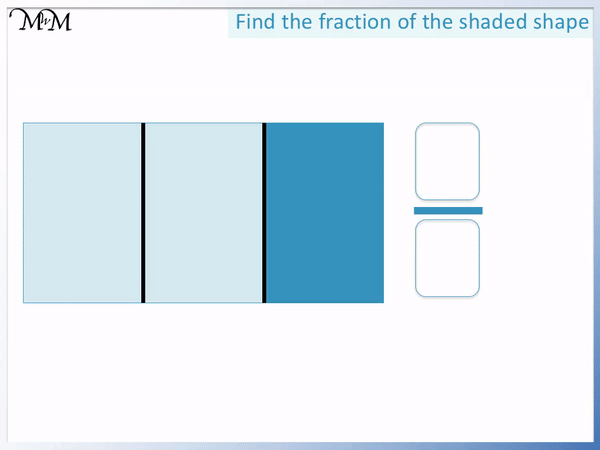To identify the fraction of a shaded shape, use these steps:

1. Count how many equal parts the shape is divided into in total.
2. Write this number as the denominator on the bottom of the fraction.
3. Draw the dividing line of the fraction above this number.
4. Count how many parts are shaded in.
5. Write this number as the numerator on the top of the fraction.

There are 4 parts in total, so the denominator is written as 4.

3 parts are shaded in, so the numerator is 3.

The fraction shown is written as   1 / 3  .

It does not matter where the parts are coloured in. When writing a fraction, it only matters how many parts there are and how many are coloured in.

Here is an example of writing the fraction of this coloured shape. We are asked to fill in the blanks of the fraction for this question.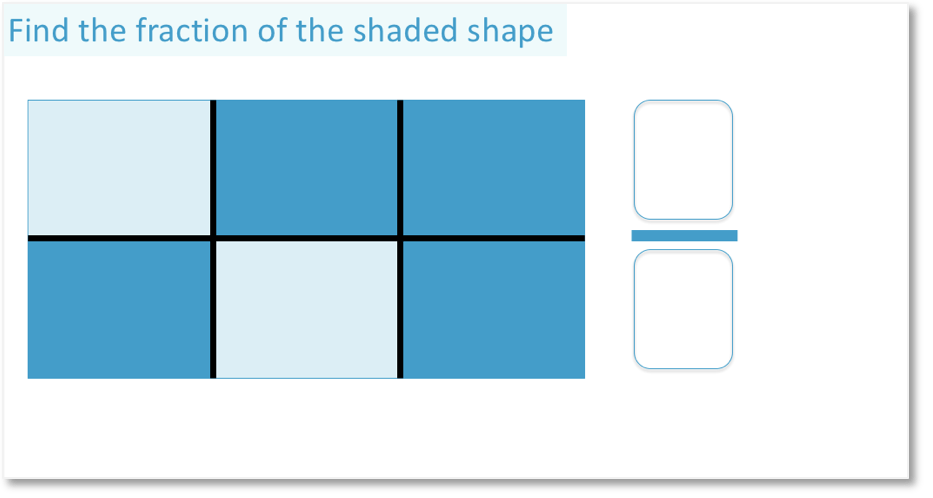Counting the number of parts, the shape is divided into 6 equally sized parts.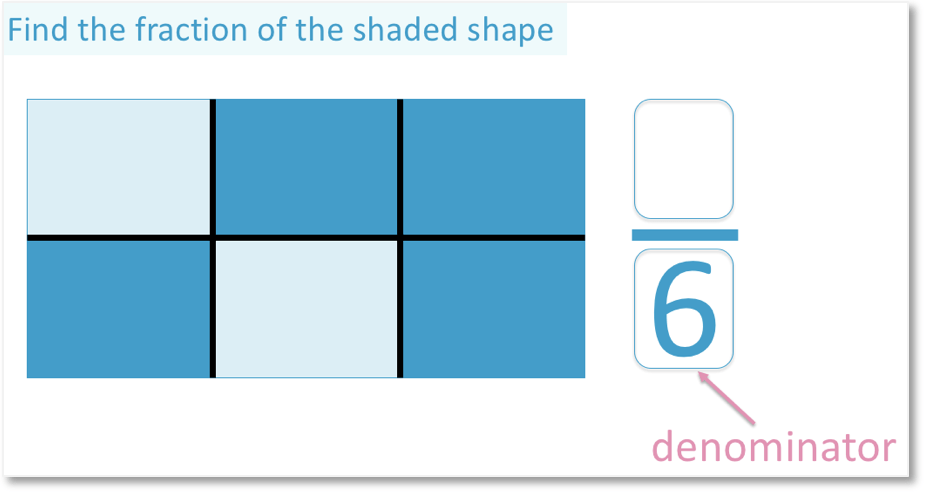We write 6 on the bottom of our fraction as the denominator below the dividing line.

In total, four parts are shaded in so a 4 is written on top of the fraction as its numerator.Four parts out of six parts are shaded in and so the fraction that is shaded is   4 / 6  . This fraction is identified as four out of 6 or four sixths.Now try our lesson on Adding Fractions with Common Denominators where we learn how to add two fractions that have the same denominator.